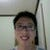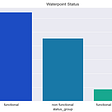# Time Series Prediction (feat. Introduction of Julia)

Continuing on the work of my previous post, I apply the similar ML model on the prediction of Bitcoin ETF. This time, I will use Julia instead of Python to code the model.

# Introduction to Julia

Julia is another popular language for Scientific Calculation. It is a function language with strict typing, contrast to the dynamic typing of Python. Although the strict typing cause some inconvenience in coding, it brings much faster speed in computation. You can try the installation of Julia by just executing the installer downloaded from its page.

# Model Assumption

Assuming the Bitcoin Trust (GBTC) is correlated to the price of Bitcoin (BTC) and Foreign Exchange Rate (FX), we can use the historical rate of return of BTC and FX rate to predict the future return of GBTC of the next day.

# Data Collection

1. Historical data of BTC can be retrieved by API (bitdataset.com).
`http://api.bitdataset.com/v1/ohlcv/history/BITFINEX:BTCUSD`

Julia provided the HTTP package to call HTTP endpoint (API endpoint). To use the package, just need the following lines of code to get the json response.

`using HTTPusing JSONraw_response = HTTP.request("GET", url_query, headers)rsp = JSON.parse(String(raw_response.body))`

Julia also provided the data structure — DataFrame similar to the family Python Pandas DataFrame. Each row retrieved from the json response above can be inserted into the DataFrame. The “!” after the function push means the function will update the variable df.

`using DataFramesdf = DataFrame(high = Float32[],low = Float32[],open = Float32[],close = Float32[],volume = Float32[], time = String[])    for d in rsp    push!(df,d)end`

2. Historical rate of FX can be retrieved by API (exchangeratesapi.io) in similar way.

`https://api.exchangeratesapi.io/history`

3. Historical stock quote of GBTC can by downloaded by Yahoo! Finanace API (yfinance). Since it is the python package, we use PyCall in Julia to call python package.

`using PyCallyf = pyimport("yfinance")ticker = yf.Ticker("gbtc")etf = ticker.history(period="3y")`

The object “etf” returned by the pycall method (ticker.history) is DataFrame in the original Python package. The Python DataFrame can be converted into Julia DataFrame.

`function pd_to_df(df_pd)    df= DataFrame()    for col in df_pd.columns        df[!, col] = getproperty(df_pd, col).values    end    df[!,:Date] = collect(df_pd[:index])    return dfendetf = pd_to_df(etf)`

# DataFrame

In Julia, DataFrame can be manipulated in the similar way as in Python.

• Apply transformation on the column, which is similar to Pandas DataFrame method: apply. Below is the transformation of the column “datestr” to Date object. The “.” after the function “Date” means the function apply on each element of the array x.
`transform!(df, :datestr => ( x -> Date.(x, Dates.DateFormat("yyyy-mm-dd")) ) => :date)`
• Read/Write CSV file for the DataFrame is simple
`using CSV    rawdata = CSV.File(infile)rawdata = DataFrame(rawdata)CSV.write(infile, rawdata)`
• Table join can be easily performed by innerjoin, and then sort by column “date”. The result is updated to “df” in place as indicated by the “!” after sort.
`df = innerjoin(df, etf, on = :date)sort!(df, [:date])`
• Pivot table can be constructed by table join as follows. Here we need to convert the FX table of all 33 countries (“Date”,”Currency”, “Rate”) to the pivot table with the currency of each country as a separated column.
1. Select the data for each kind of currency (“c”) by using the Package “DataFramesMeta” and “@linq”
`using DataFramesMetadf1 = @linq rawdata |> where(:CUR .== c) |> select(:ondate,:rate_mean)`

2. Join the above output with the “on-date” column.

`coldata = leftjoin(datecol,df1,on=:ondate)`

3. Assign the result as the column data for that currency “c”.

`df[:,c] = coldata[:,:rate_mean]`
`df = DataFrame()df.ondate = unique(rawdata.ondate)datecol = dffor c in unique(rawdata.CUR)    df1=@linq rawdata|>where(:CUR .== c)|>select(:ondate,:rate_mean)    coldata = leftjoin(datecol,df1,on=:ondate)    df[:,c] = coldata[:,:rate_mean]end`

Above is the full for-loop to convert to the following pivot table.

• Finally, cleanse the data by dropping the rows with missing data.
`dropmissing!(df)`

# Feature Engineering

• I compute the rate of return from the FX rate and price quote of BTC and GBTC using the Julia package TimeSeries. The function “percentchange” can easily compute the change of the current value over the lag-value.
• Then, apply PCA on all the input columns (FX rates of 33 countries, and the BTC open, close,high,low daily price, and its daily transaction volume). Compute the first 3 PCA components as the final input variables.
• Concatenate current and previous values of the 3 PCA output from time i to i+seqlen-1 (pcax[:,i:i+seqlen-1])
• Prediction target is the GBTC close price return of the next day at time=i+seqlen (df[i+seqlen,target])
`ts = TimeArray(df,timestamp=:date)pct = percentchange(ts)df = DataFrame(pct)mx = transpose(convert(Matrix,df[:,features]))M = MultivariateStats.fit(PCA, mx; maxoutdim=3)pcax = MultivariateStats.transform(M, mx)for i in 1:len-seqlen-1        x = pcax[:,i:i+seqlen-1]        xtrain = vcat(xtrain,[x])        y = df[i+seqlen,target]        ytrain = vcat(ytrain,[y])end`

# Neural Network Model

We stack Convolution Layer over Recurrent Layer GRU.

Flux is the ML package for Julia to code Neutral Network.

• Since the input shape of Convolution layer is 4-D, we need to append 2 more dimensions using unsqueeze.
• After 2 layers of Conv/MaxPool, we flatten all the channel output.
• Then, transpose it in order to match with the GRU input layer.
• As I only concern the prediction of the last time step, we append the layer (x -> x[:end]) to the output of the GRU layer.
• Finally, we append the dense layer to get the final prediction.
`using Fluxfunction build_model(Nh)    a = floor(Int8,Nh)    return Chain(    x -> Flux.unsqueeze(Flux.unsqueeze(x,3),4),        # First convolution    Conv((2, 2), 1=>a, pad=(1,1), relu),    MaxPool((2,2)),    # Second convolution    Conv((2, 2), a=>Nh, pad=(1,1), relu),    MaxPool((2,2)),    Flux.flatten,    Dropout(0.1),    (x->transpose(x)),    GRU(1,Nh),    GRU(Nh,Nh),    (x -> x[:,end]),    Dense(Nh, 1),    (x -> x))end`

# Training Result

Train the model “m” as follows:

`train_loader = Flux.Data.DataLoader(xtrain,ytrain, batchsize=batchsize,shuffle=false)m = build_model(Nh)    function mae_loss(x,y)     yh = m.(x)     e = Flux.mae(yh,y)     return eend@epochs num_epoch Flux.train!(mae_loss,Flux.params(m),train_loader,RMSProp(lr))`

The training result is not good. Here is the plot of the predicted value y1 (red) and the training data y2 (blue). The predicted value cannot fit with the actual value.

You can observe that the actual value is highly fluctuating, that may causing the Gradient Decent not able to converge. Therefore, I try to use moving averge to smoothen the data. I compute the 10-day moving average before the PCA dimension reduction. I apply the 10-day moving average on both input variable sand target variable.

`pct = percentchange(ts)ma = moving(mean, pct, 10)`

Here is the plot of the prediction y1 (red) and the training data y2 (blue) after using moving average. The prediction can well fit the actual line. Bingo!

# Test with unseen data

I re-run the training by holding out the last 250 days of data for verification and testing. The result is still good.

# Data Leakage?

For fear that the improvement of the result is caused by the data leakage in the moving average computation, I revisit the calculation in details.

First, the input variables are the values from time i to i+seqlen-1. The moving average is taking the mean of that value with the values of previous 9 days. Therefore, it will not have the future value at time i+seqlen. Although I also take the moving average for the target variable, the input still does not contain the future value at time i+seqlen.

To play safe, I try to run the same model on other target data. If the good result is caused by data leakage instead of the data correlation, I would get the similar result for other target data. I tried the stocks: SQ, GLD, and 2840.HK. SQ is a NASAQ stock related to Bitcoin. GLD and 2840 are the Gold ETF in US market and HK market. Here is the fitting of the training data. The result showed that target data with less correlation cannot fit into the model well even after using moving average.

# Comment on Julia

Although Julia is claimed to be very fast in the computation, the compilation overhead will make the code slow to run at the first time. It will take some time for pre-compilation when you import the packages with the command “using <pkg>”.

Besides, it is not easy to use because of the strict typing. Especially the DataFrame is not as handy as Python Pandas DataFrame.

You can checkout the full Julia code in my git repo (module file / notebook).

--

--

--

## More from Analytics Vidhya

Analytics Vidhya is a community of Analytics and Data Science professionals. We are building the next-gen data science ecosystem https://www.analyticsvidhya.com

## Mutation Testing: Quis Custodiet Ipsos Custodes## Entrepreneurs — Make Mondays Great Again## Merge Sort: How To Understand an Algorithm by Creating Music## Actually, Agile isn’t that great sometimes## Integrating Mpesa API with a Flutter App## Unity-Up and Running## Tanzanian Waterpoints: Ternary classification with three ML models## Anomaly Detection using LSTM Autoencoder## The Reasonable Effectiveness of Deep Learning for Time Series Forecasting